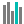#PERMUTATIONA (PQL - xl)

This function calculates the number of permutations for the specified number of items (along with repetitions) that can be selected from the total items

• Library: PQL \ Spreadsheet \ Statistical
• Compatibility: Any content (regardless of data source) in the Tabulate spreadsheet module

#### Syntax

PERMUTATIONA(number, number chosen)

##### Function Arguments
 Name Description Type Optional number Refers to the total number of items. This value must be greater than 0. If the specified value is not an integer, the number is truncated Number number chosen Refers to the number of items in each possible permutation. This value must be positive or 0. If the specified value is not an integer, the number is truncated Number